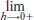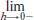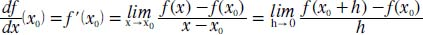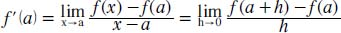NextPrevious

# What is the standard notation for the derivative?

The following represents the definition of the derivative of f(x) (note: in order for the limit to exist, bothand—must exist and be equal; thus, the function must be continuous):

For f (x)’s derivative at point x0:For f(x)’s derivative at x = a:Close

This is a web preview of the "The Handy Math Answer Book" app. Many features only work on your mobile device. If you like what you see, we hope you will consider buying. Get the App